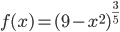### Day 41 - Optimization - 10.14.15

Update

Bell Ringer
1. Find all of the critical numbers for.

1. –3, 0, 3

2. 3

3. 3, –3

4. 0

5. none of the above

2. Locate the absolute extrema of the functionon the closed interval [–6, 6]

1. No absolute max, Absolute min:2. Absolute max:, Absolute min:3. Absolute max:, No absolute min

4. Absolute max:, Absolute min:5. No absolute max or min

3. Determine whether Rolle's Theorem can be applied to the functionon the closed interval [–1, 5].  If Rolle´s Theorem can be applied, find all values of c in the open interval (–1, 5) such that.

1. Rolle’s Theorem applies;  c = –2

2. Rolle’s Theorem applies;  c = 0.5

3. Rolle’s Theorem does not apply

4. Rolle’s Theorem applies;  c = 2

5. both a and d

4. Determine whether the Mean Value Theorem can be applied to the functionon the closed interval [0, 16]. If the Mean Value Theorem can be applied, find all numbers c in the open interval (0, 16) such that.

1. MVT applies;  c = 4

2. MVT applies;  c =3. MVT applies;  c = 8

4. MVT applies;  c =5. MVT does not apply

5. Find all intervals on whichis concave upward.

1.2.3.4.5. none of these
• Pre-calculus
• Extrema
• Minima
• Maxima
• Absolute
• Relative
• Interval Notation
• Extrema (video) (checkpoints)
• How can extrema be defined for a function?
• How can critical numbers be calculated using derivatives?
• How are critical numbers related to extrema?
• Absolute and Relative Extrema (video)
• Critical Numbers (video)
• Increasing/Decreasing Functions (video) (checkpoints)
• How are derivatives related to functions increasing and decreasing?
• Rolle's Theorem & Mean Value Theorem
• How are Rolle's Theorem and Mean Value Theorem related to differentiation?
• Concavity and Inflection Points
• How are derivative related to intervals of concave upwards and downwards?

Lesson

• Posted on the board at the end of the block

Homework
• N/A

#### In-Class Help Requests

Standard(s)
• APC.7
• Analyze the derivative of a function as a function in itself.
• Includes:
• comparing corresponding characteristics of the graphs of f, f', and f''
• ​defining the relationship between the increasing and decreasing behavior of f and the sign of f'
• ​translating verbal descriptions into equations involving derivatives and vice versa
• analyzing the geometric consequences of the Mean Value Theorem;
• defining the relationship between the concavity of f and the sign of f"; and ​identifying points of inflection as places where concavity changes and finding points of inflection.
• APC.8
• Apply the derivative to solve problems.
• Includes:
• ​analysis of curves and the ideas of concavity and monotonicity
• optimization involving global and local extrema;
• modeling of rates of change and related rates;
• use of implicit differentiation to find the derivative of an inverse function;
• interpretation of the derivative as a rate of change in applied contexts, including velocity, speed, and acceleration; and
• differentiation of nonlogarithmic functions, using the technique of logarithmic differentiation.*
• *AP Calculus BC will also apply the derivative to solve problems.
• Includes:
• ​analysis of planar curves given in parametric form, polar form, and vector form, including velocity and acceleration vectors;
• ​numerical solution of differential equations, using Euler’s method;
• ​l’Hopital’s Rule to test the convergence of improper integrals and series; and
• ​geometric interpretation of differential equations via slope fields and the relationship between slope fields and the solution curves for the differential equations.